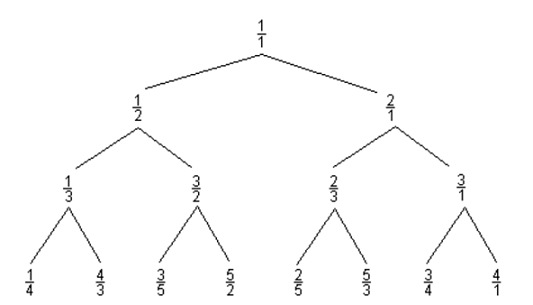# Perl Weekly Challenge 146.

My solutions (task 1 and task 2 ) to the The Weekly Challenge - 146.

# Task 1: 10001st Prime Number

``````Submitted by: Mohammad S Anwar
Write a script to generate the 10001st prime number.
``````

We can borrow a canned solution, which yields a trivial oneliner.

``````perl -MMath::Prime::Util=nth_prime -E 'say nth_prime(10001)'
``````

Results:

``````104743
``````

A somewhat less cynical solution may be obtained by running an Erastothenes sieve on a large enough array and choosing the 10001’st term, which may be very compactly stated using the Perl Data Language (PDL).

``````perl -MPDL -MPDL::NiceSlice -E '\$s=ones(120000);
\$s(0:1).=0; \$s(\$_*\$_:-1:\$_).=0 for(2..sqrt(120000-1));
\$p=\$s->xvals->where(\$s); say \$p((10001-1))'
``````

Here `\$s` is a sieve, initialized to 1’s and set to zero for all non-trivial multiples of previous numbers, and `\$p` is the array of primes. The result is:

``````104743
``````

A more general solution follows, which takes as an optional argument the desired prime’s position.

`````` 1  # Perl weekly challenge 146
2  # Task 1: 10001st prime
3  #
5  use v5.12;
6  use warnings;
7  use PDL;
8  use PDL::NiceSlice;
9  use Try::Tiny;
10  for my \$N(@ARGV?@ARGV:10001){
11      try{
12          die "Argument \$N is not positive\n" unless \$N>=1;
13          # Estimate size \$M of required sieve by solving \$M/log(\$M) approx \$N
14          # unless \$N is too small
15          my \$M=\$N<4?6:find_zero(sub {my \$x=shift; \$N-\$x/log(\$x)},
16                          sub {my \$l=log(\$_); 1/\$l**2-1/\$l}, \$N);
17          my \$sieve=ones(\$M); # fill sieve with ones
18          \$sieve(0:1).=0; # 0 and 1 are not primes
19          \$sieve(\$_*\$_:-1:\$_).=0 foreach(2..sqrt(\$M-1)); # multiples of 'it' are not prime
20          my \$primes=\$sieve->xvals->where(\$sieve); # first primes
21          die "Short sieve" unless \$N<=\$primes->nelem; # shouldn't happen
22          my \$Nth=\$N>1?"\$N-th":"\$N-st";
23          say "The \$Nth prime is ", \$primes((\$N-1));
24      }
25      catch { say \$_;}
26  }
27
28  no PDL::NiceSlice; # NiceSlice destroys indirect function calls!
29  sub find_zero { # Find zero of function using Newton's iteration
30      my (\$f, \$d, \$x0)=@_; # function, derivative, initial guess
31      my \$x=\$x0;
32      my \$y;
33      my \$max=10; # guard against non-convergence
34      do{(\$y, \$x)= (\$x, \$x-\$f->(\$x)/\$d->(\$x))} until approx(\$y,\$x) or --\$max<=0;
35      die "find_zero didn't converge starting from \$x0\n" unless \$max>0;
36      return \$x;
37  }
38
``````

Example:

``````./ch-1.pl
``````

Results:

``````The 10001-th prime is 104743
``````

Other examples:

``````./ch-1.pl 11 101 1001 10001 100001
``````

Results:

``````The 11-th prime is 31
The 101-th prime is 547
The 1001-th prime is 7927
The 10001-th prime is 104743
The 100001-th prime is 1299721
``````

Example with an error:

``````./ch-1.pl 0 1
``````

Results:

``````Argument 0 is not positive

The 1-st prime is 2
``````

# Task 2: Curious Fraction Tree

``````Submitted by: Mohammad S Anwar
Consider the following Curious Fraction Tree:
````````````You are given a fraction, member of the tree created similar
to the above sample.

Write a script to find out the parent and grandparent of the
given member.

Example 1:
Input: \$member = '3/5';
Output: parent = '3/2' and grandparent = '1/2'
Example 2:
Input: \$member = '4/3';
Output: parent = '1/3' and grandparent = '1/2'
``````

The tree grows by adding the numerator and denominator and substituting the result for the denominator (left branches) and for the numerator (right branches). Thus, consider `n/m`. Its parent should be `(n-m)/m` if `m<n`, and `n/(m-n)` if `n<m`. A simple solution is the one-liner

``````perl -E 'sub p{my (\$n,\$d)=@_; return \$d>\$n?(\$n,\$d-\$n):(\$n-\$d,\$d)} @gp=p(@p=p(@ARGV));
say "Input: \$ARGV/\$ARGV, Parent: \$p/\$p, Grandparent: \$gp/\$gp"' 3 5
perl -E 'sub p{my (\$n,\$d)=@_; return \$d>\$n?(\$n,\$d-\$n):(\$n-\$d,\$d)} @gp=p(@p=p(@ARGV));
say "Input: \$ARGV/\$ARGV, Parent: \$p/\$p, Grandparent: \$gp/\$gp"' 4 3
``````

Results:

``````Input: 3/5, Parent: 3/2, Grandparent: 1/2
Input: 4/3, Parent: 1/3, Grandparent: 1/2
``````

If `n=m` then we are already at the root of the tree. The tree only contains reduced fractions, so I might have to cancel out common factors. Thus a full solution would be:

`````` 1  # Perl weekly challenge 146
2  # Task 2: Curious Fraction Tree
3  #
5  use v5.12;
6  use warnings;
7  use Try::Tiny;
8  foreach(@ARGV){
9      try {
10          my(\$n, \$d)=(\$1,\$2) if m{^\s*(\d+)\s*/\s*(\d+)\s*\$};
11          die "Wrong argument \$_\n" unless defined \$n and defined \$d;
12          die "Numerator and denominator in \$_  should be positive\n"
13              unless \$n>0 and \$d>0;
14          my \$gcd=gcd(\$n, \$d);
15          say "Warning: \$_ not reduced" unless \$gcd==1;
16          (\$n, \$d)=map {\$_/\$gcd} (\$n, \$d);
17          my @parent=parent(\$n, \$d);
18          try {
19              my @grand_parent=parent(@parent);
20              say "Input: \$n/\$d\nParent:\$parent/\$parent\n",
21                  "Grand parent: \$grand_parent/\$grand_parent\n";
22          }
23          catch {
24              die "No grandparent of \$n/\$d\n";
25          }
26      }
27      catch {
28          say \$_;
29      }
30  }
31
32  sub gcd {
33      my (\$n, \$m)=@_;
34      (\$n, \$m)=(\$m, \$n%\$m) while (\$m);
35      return \$n;
36  }
37  sub parent {
38      my (\$n, \$d)=@_;
39      die "No parent of \$n/\$d\n" if \$n==\$d;
40      return \$d>\$n?(\$n,\$d-\$n):(\$n-\$d,\$d);
41  }
42
``````

I used the try/catch mechanism from `Try::Tiny` module to recover from errors when present.

Examples:

``````./ch-2.pl 3/5 4/3
``````

Results:

``````Input: 3/5
Parent:3/2
Grand parent: 1/2

Input: 4/3
Parent:1/3
Grand parent: 1/2
``````

Some examples with errors:

``````./ch-2.pl 0/1 1/1 1/2 24/16 22/22 2 2.5/4.3
``````

Results:

``````Numerator and denominator in 0/1  should be positive

No parent of 1/1

No grandparent of 1/2

Warning: 24/16 not reduced
Input: 3/2
Parent:1/2
Grand parent: 1/1

Warning: 22/22 not reduced
No parent of 1/1

Wrong argument 2

Wrong argument 2.5/4.3
``````
Written on January 3, 2022